# Chapter 12 Surface Areas and Volumes NCERT Exemplar Solutions Exercise 12.3 Class 10 Maths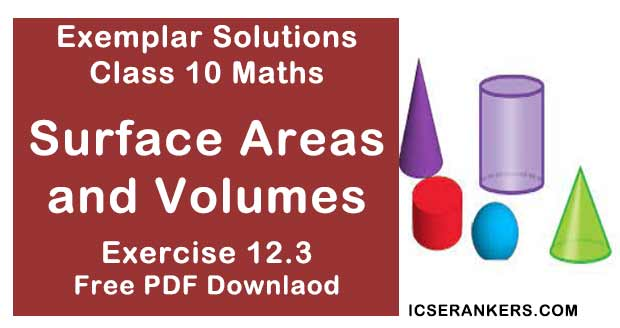Chapter Name NCERT Maths Exemplar Solutions for Chapter 12 Surface Areas and Volumes Exercise 12.3 Book Name NCERT Exemplar for Class 10 Maths Other Exercises Exercise 12.1Exercise 12.2Exercise 12.4 Related Study NCERT Solutions for Class 10 Maths

### Exercise 12.3 Solutions

1. Three metallic solid cubes whose edges are 3 cm, 4 cm and 5 cm are melted and formed into a single cube. Find the edge of the cube so formed.

Solution

We know that,
Volume of cube = a3 ,
According to the question,
Side of first cube,
a1 = 3 cm
Side of second cube,
a2 = 4 cm
Side of third cube,
a3 = 5 cm
Let us assume that the side of cube recast from melting these cubes = a
We know that the total volume of the 3 cubes will be the same as the volume of the newly formed cube,
Volume of new cube = (volume of 1st + 2nd + 3rd cube)
a3 = a13 + a23 ,
⇒ a3 = (3)3 + (4)3 + (5)3 ,
⇒ a3 = 27 + 64 + 125
⇒ a3 = 216
⇒ a = 6 cm
Therefore, side of cube so formed is 6 cm.

2. How many shots each having diameter 3 cm can be made from a cuboidal lead solid of dimensions 9cm × 11cm × 12cm?

Solution

Volume of cuboid = lbh,
Where, l = length, b = breadth and h = height
Length, l = 9 cm
Height, h = 12 cm
Volume of lead = 9(11) (12)
= 1188 cm3
Volume of sphere = (4/3)πr3 , where r = radius of sphere
Spherical shots,
Diameter = 3 cm
Volume of one shot  = (4/3) × (22/7) × (1.5)3
= 99/7  cm3
No. of shots can be made = (Volume of lead)/(Volume of one shot)
= 1188/99/7 = 84
Hence, the number of bullets that can be made from lead = 84.

3. A bucket is in the form of a frustum of a cone and holds 28.490 litres of water. The radii of the top and bottom are 28 cm and 21 cm, respectively. Find the height of the bucket.

Solution

According to the question,
The bucket is in the form of frustum of a cone.
We know that,
Volume of frustum of a cone = (1/3)πh(r12 + r22 + r1r2),
Where, h = height, r1 and r2 are the radii (r1 > r2)
For bucket,
Volume of bucket = 28.490 L
1 L = 1000 cm3
Volume of bucket = 28490 cm3
Radius of top, r1 = 28 cm
Radius of bottom, r2 = 21 cm
Let the height = h.
Substituting these values in the equation to find the volume of bucket,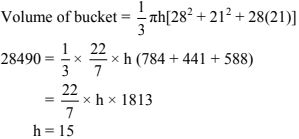4.  A cone of radius 8 cm and height 12 cm is divided into two parts by a plane through the mid-point of its axis parallel to its base. Find the ratio of the volumes of two parts.
SolutionAccording to the question,
Height of cone = OM = 12 cm
The cone is divided from mid-point.
Hence, let the mid-point of cone = P
OP = PM = 6 cm
From △OPD and △OMN
∠POD = ∠POD [Common]
∠OPD = ∠OMN [Both 90°]
Hence, by the Angle-Angle similarity criterion
We have,
△OPD ~ △OMN
and
Similar triangles have corresponding sides in equal ratio,
So,
PD/MN = OP/OM
PD/8 = 6/12
PD = 4 cm
[MN = 8 cm = radius of base of cone]
For First part i.e. cone
r = PD = 4 cm
Height,
h = OP = 6 cm
We know that,
Volume of cone for radius r and height h,
V = (1/3)πr2 h
Volume of first part  = (1/3)π(4)(26)
= 32π
For second part, Frustum,
r1 = MN = 8 cm
r2 = PD = 4 cm
Height,
h = PM = 6 cm
We know that,
Volume of frustum of a cone = (1/3)πh(r12 + r22 + r1r2),
Where, h = height , r1 and r2 are radii,
Volume of second part  =  (1/3)π(6)[82 + 42 + 8 × 4],
= 2π(112)
= 224π
Therefore, we get the ratio,
Volume of first part : Volume of second part
32π : 224π
1 : 7

5. Two identical cubes each of volume 64 cm3  are joined together end to end. What is the surface area of the resulting cuboid?
Solution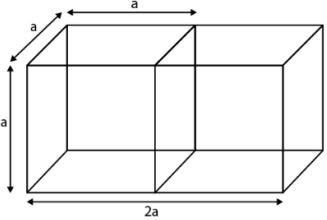Let the side of one cube = a
Surfaces area of resulting cuboid = 2(Total surface area of a cube) - 2(area of single surface)
We know that,
Total surface area of cube = 6a2, Where a = side of cube
Surfaces area of resulting cuboid = 2(6a2) - 2(a2)
= 10a2
Also,
According to the question,
Volume of cube = 64 cm3
Volume of cube = a3
64 = a3
⇒ a = 4 cm
Therefore,
Surface area of resulting cuboid = 10 a2
= 10(4)2
= 160 cm2

6. From a solid cube of side 7 cm, a conical cavity of height 7 cm and radius 3 cm is hollowed out. Find the volume of the remaining solid.
Solution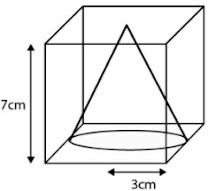From the figure, we get,
Volume of remaining solid = volume of cube – volume of cone
For Cube,
Side, a = 7 cm
We know that,
Volume of cube = a3, where a = side of cube
Volume of cube = (7)3
= 343 cm3
For cone,
Height, h = 7 cm
Volume of cone  = (1/3)πr2 h
= (1/3)π(3)3 (27)
= 3 × (22/7) × 7
= 66 cm3
Volume of remaining solid  = Volume of cube - Volume of cone
= 343 - 66
= 277 cm3

7. Two cones with same base radius 8 cm and height 15 cm are joined together along their bases. Find the surface area of the shape so formed.
Solution
According to the question,
We get the figure given below,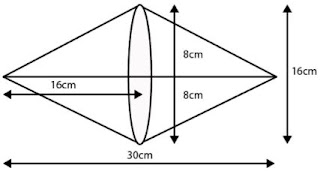Total surface area of shape formed = Curved area of first cone + Curved surface area of second cone
Since, both cones are identical,
Total surface area of shape formed = Curved area of first cone + Curved surface area of second cone
= 2(Surface area of cone)
We also know that,
Surface area of cone = πrl, where r = radius and l = slant height
The total Surface area of shape so formed = 2πrl
Given in the question that,
Height, h = 15 cm
Therefore,
Area = 2(3.14)(8)(15)
= 753.6 cm2

8.Two solid cones A and B are placed in a cylindrical tube as shown in the Fig. The ratio of their capacities are 2:1. Find the heights and capacities of cones. Also, find the volume of the remaining portion of the cylinder.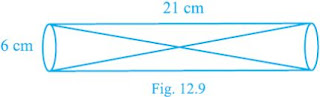Solution
Let volume of cone A be 2 V and volume of cone B be V.
Again, let height of the cone A = h1 cm, then height of cone  B = (21 - h1 ) cm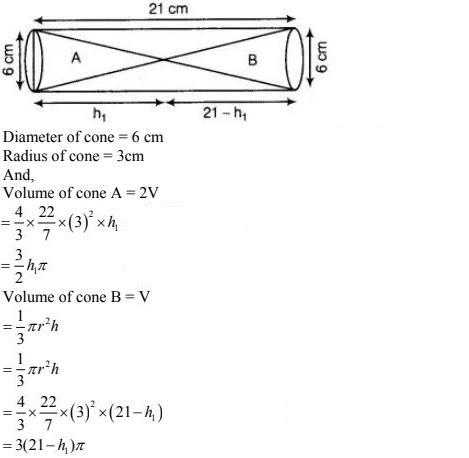According to question,
(3/2)h1 π = 3(21 - h1 )π
h1 = 14 cm
So,
Height of cone B = 21 - h1
= 7 cm
Volume of cone A = (3/2)h1 π
= 132 cm3
Volume of cone B = 3(21 - h1 )π
= 66 cm3
Volume of cylinder = πr2 h
= 594 cm3
Volume of remaining portion  = Volume of cylinder - (Volume of cone A + Volume of cone B)
= 594 - (132 + 66)
= 396 cm3

9. An ice cream cone full of ice cream having radius 5 cm and height 10 cm as shown in the Fig.12.10. Calculate the volume of ice cream, provided that its 1/6 part is left unfilled with ice cream.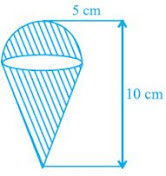Solution
Given, ice - cream cone is the combination of a hemisphere and a cone .
Also, radius of hemisphere = 5 cm
Volume  of hemisphere,
= (4/3)πr3
= (4/3)× 22/7 × (5)3
= 5500/21
= 261.90 cm3
Now,
Height of cone  = 5
Volume of cone,
= (1/3) πr2 h
= (4/3) × 22/7 × (5)2 × 5
= 130.95 cm3
Total volume of ice - cream cone = 261.90 + 130.95
As,
1/6 part is left unfilled,
Required volume of ice - cream = 392.85 - 392.85 × 1/6
= 327.4 cm3

10. Marbles of diameter 1.4 cm are dropped into a cylindrical beaker of diameter 7 cm containing some water. Find the number of marbles that should be dropped into the beaker so that the water level rises by 5.6 cm.
Solution
Given,
Diameter of a marble = 1.4 cm
Volume of spherical marble = (4/3)πr3
= (4/3) × (22/7) × (0.7)3
= (1.372/3)π  cm3
Diameter of beaker = 7 cm
Height of water raised  = 5.6 cm
Volume of water raised in beaker,
= π(3.5)2 × 5.6
= 68.6 π
Required no. of marbles = (Volume of water raised in beaker)/(Volume of marble)
= 150

11. How many spherical lead shots each of diameter 4.2 cm can be obtained from a solid rectangular lead piece with dimensions 66 cm, 42 cm and 21 cm.
Solution
Number of spherical lead shots = (Volume of solid rectangular lead piece)/(Volume of spherical lead shot )
Diameter of s spherical lead shot = 4.2 cm
Now,
Volume of solid rectangular lead piece = 46× 42 × 21
Volume of spherical lead shot  = (4/3)πr3
= (4/3) × 22/7 × (2.1)3
= (4 × 22 × 2.1 × 2.1 × 2.1)/21
= 1500
Hence, the required number of spherical lead shots is 1500.

12. How many spherical lead shots of diameter 4 cm can be made out of a solid cube of lead whose edge measures 44 cm.
Solution
Diameter of a spherical lead shot = 4cm
Now,
Volume of solid cube = 44 × 44 × 44
Volume of spherical lead shot = (4/3)πr3
= (4/3) × (22/7) × 23
= (4 × 22 × 8)/21
= 2541
Hence, the required number of spherical lead shot is  2541.

13. A wall 24 m long, 0.4 m thick and 6 m high is constructed with the bricks each of dimensions 25 cm × 16 cm × 10 cm. If the mortar occupies (1/10)th of the volume of the wall, then find the number of bricks used in constructing the wall.
Solution
Given that, a wall is constructed with the help of bricks and mortar.
Number of bricks = (Volume of wall - 1/10 volume of wall)/(Volume of a brick)
Given,
Length of wall = 24
Height of wall = 6
Now,
Volume of wall constructed with bricks = 24 × 0.4 × 6 m3
So,
1/10 Volume of wall = 1/10 × 24 × 0.4 × 6
Also,
Length of brick = 25 cm
Height of brick = 10cm
Volume of bricks = 0.25 × 0.16 × 0.10 m3
So,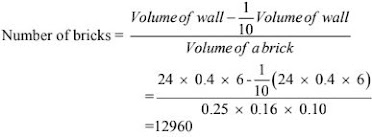14.  Find the number of metallic circular disc with 1.5 cm base diameter and of height 0.2 cm to be melted to form a right circular cylinder of height  10 cm and diameter 4.5 cm.
Solution
Given that, lots of metallic circular disc to be melted to form a right circular cylinder.
Here, a circular disc work as a circular cylinder.
Base diameter of metallic circular disc = 1.5 cm